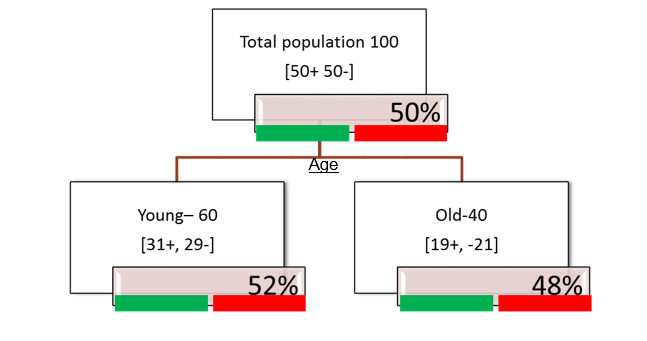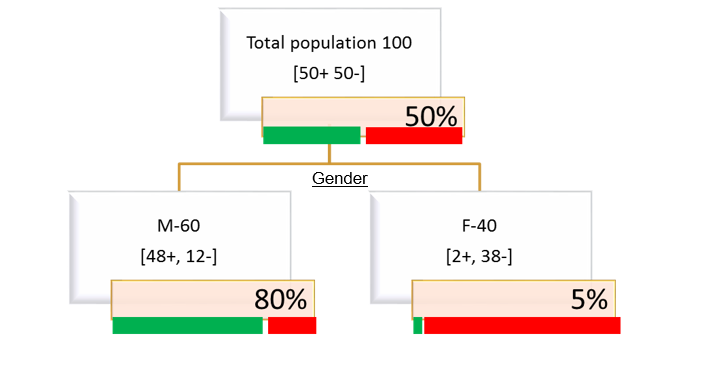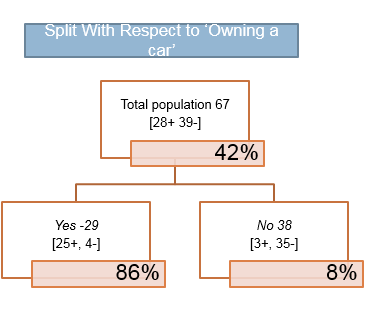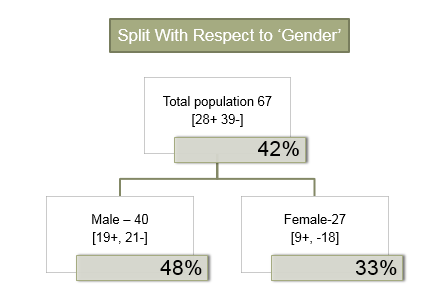• No products in the cart.

# 204.3.5 Information Gain in Decision Tree Split

##### Calculating information gain after a split in Decision Tree algorithm.

Link to the previous post : https://statinfer.com/204-3-4-how-to-calculate-entropy-for-decision-tree-split/

In the previous post of this series we calculated the entropy for each split. In this post we will calculate the information gain or decrease in entropy after split.

## Information Gain

• Information Gain= entropyBeforeSplit – entropyAfterSplit.
• Easy way to understand Information gain= (overall entropy at parent node) – (sum of weighted entropy at each child node).
• Attribute with maximum information is best split attribute.

### Information Gain- Calculation• Entropy Ovearll = 100% (Impurity)
• Entropy Young Segment = 99%
• Entropy Old Sgment = 99%
• Information Gain for Age =100-(0.699+0.499)=1• Entropy Ovearll = 100% (Impurity)
• Entropy Male Segment = 72%
• Entropy Female Sgment = 29%
• Information Gain for Age =100-(0.672+0.429)=45.2

### Practice : Information Gain

Calculate the information gain this example base on the variable split### Output-Information Gain

Split With Respect to ‘Owning a car’

• Entropy([28+,39-]) Ovearll = -28/67 log2 28/67 – 39/67 log2 39/67 = 98% (Impurity)
• Entropy([25+,4-]) Owing a car = 57%
• Entropy([3+,35-]) No car = 40%
• Information Gain for Owing a car =98-((29/67)57+(38/67)40)=50.6

Split With Respect to ‘Gender’

• Entropy([19+,21-]) Male= 99%
• Entropy([9+,18-]) Female = 91%
• Information Gain for Gender=98-((40/67)99+(27/67)91) =2.2

## Other Purity (Diversity) Measures

• Chi-square measure of association
• Gini Index : Gini(T) = 1p2j
• Information Gain Ratio
• Misclassification error

The next post is about the decision tree algorithm.

Link to the next post : https://statinfer.com/204-3-6-the-decision-tree-algorithm/

21st June 2017
0 responses on "204.3.5 Information Gain in Decision Tree Split"
© 2020. All Rights Reserved.